# Replace Negative Values with Zero in Excel & Google Sheets

In this tutorial we will demonstrate how to replace negative numbers with zeros in Excel & Google Sheets.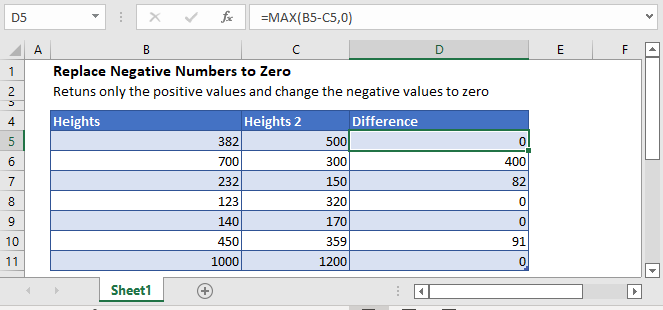## Replace Negative Numbers with Zero

Sometimes you don’t want negative values in your data. You can replace negative values with zero using one of the following three methods.

## MAX Function

The MAX Function returns the maximum value from a set of numbers. Here we will use the MAX Function to calculate the max value between 0 and a calculated value.

For example, we have a table with heights values in Column B and C. Now, we need to calculate the difference between Heights 1 and Heights 2 but would like the results to only show positive values and replace the negative values with zero. So to do that, we used the following formula:

``=MAX(B3-C3,0)``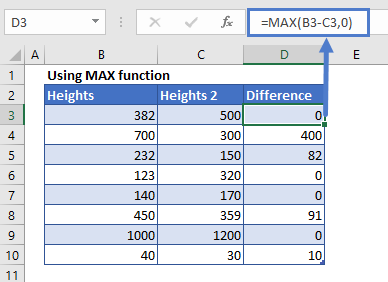## IF Function

We can also use the IF function to force negative numbers to zero. The IF function tests a condition and returns a value depending on whether the condition is true or false.

Let’s say we have a set of data in column B with positive and negative values. Now, we need only positive numbers to show in column C.

To do this, we put the following formula in Cell C3 and autofill the rest of the cells:

``=IF(B3<0,0,B3)``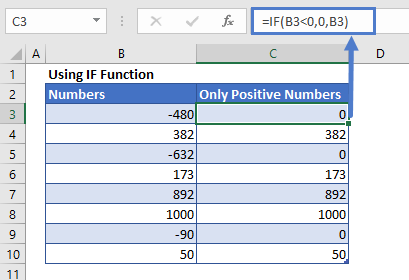## Display Negative Values as Zeros

The above two methods not only display the negative value as zero but also changes the value to zero.

Instead, we can change the number formatting to 0;”0”;0. This will display negative numbers as zero.

Note: We use this method with extreme caution. It can cause great confusion in your workbook!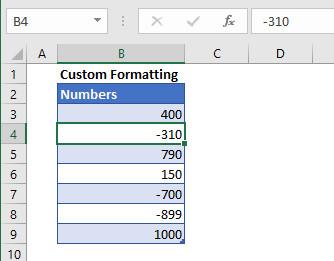### Change Number Formatting

1. Select a range of cells
2. Press CTLR+1 to access the Number Format Dialog Box.
3. Select Custom
4. Type 0;”0”;0 in the Type box and click the OK button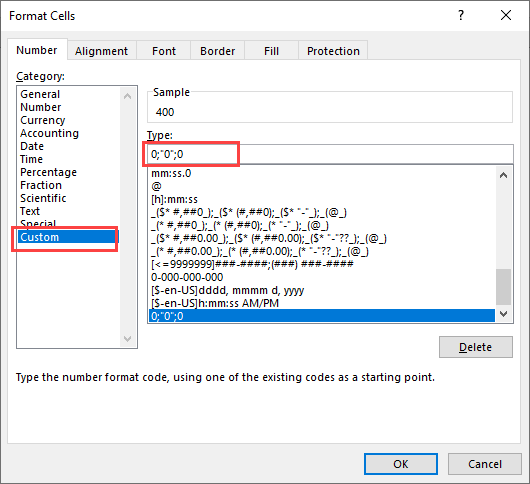This changes the display of all the negative values to zero, maintaining the original cell value.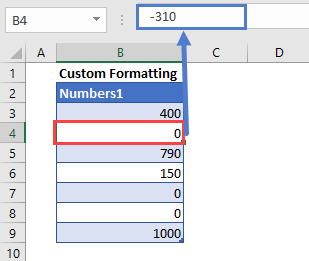## Replace Negative Numbers to Zero in Google Sheets

The formula to replace negative numbers to zero works exactly the same in Google Sheets as in Excel: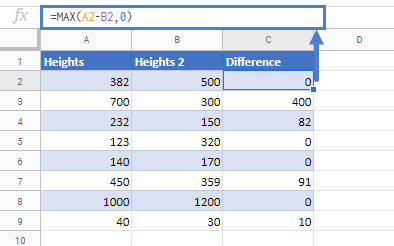### Excel Practice Worksheet

Practice Excel functions and formulas with our 100% free practice worksheets!